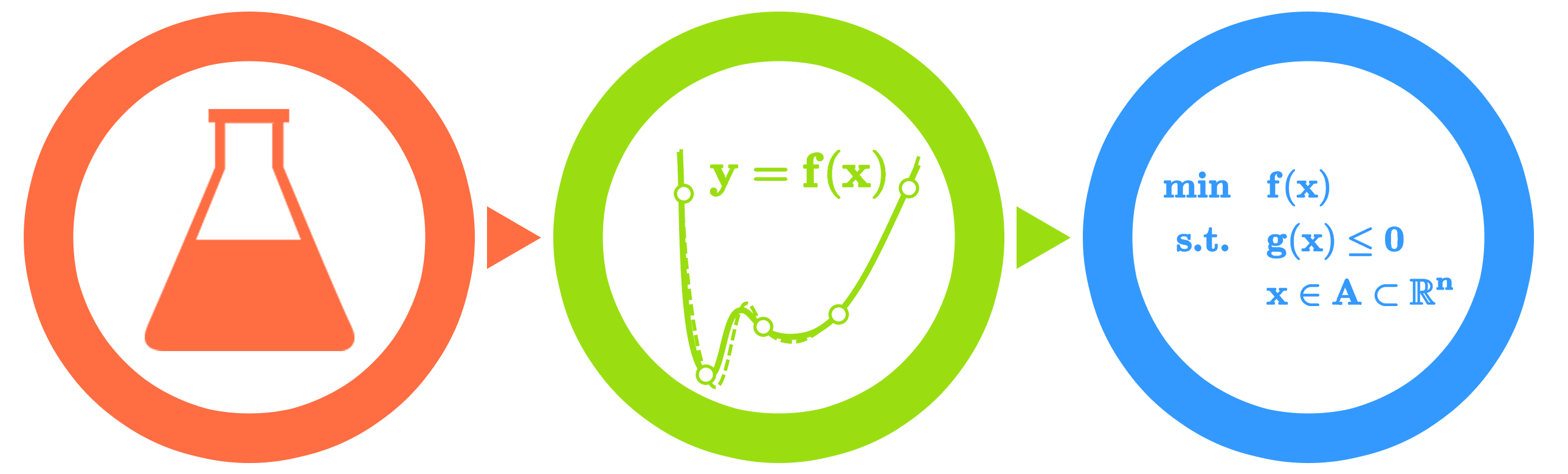# Home## Purpose

ALAMO generates algebraic models of simulations, experiments, or other black-box systems. The models provided by ALAMO are:

• accurate
• simple in functional form
• generated from a minimal experimental data set.

ALAMO uses an iterative design of experiments to build low-complexity surrogate models while answering the following questions:

• What is the functional form of the model?
• How complex of a model is needed?
• Will this be tractable in an algebraic optimization framework?
• How many sample points are needed to define an accurate model and where should they be sampled?